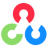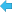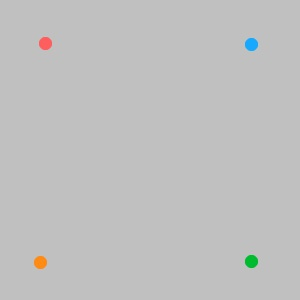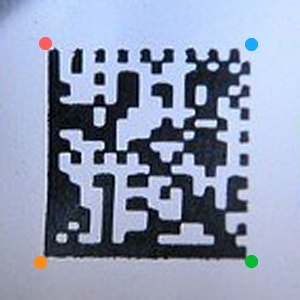Back to Adaptive Vision Studio website# cvGetPerspectiveTransform

Module: OpenCV

Calculates the perspective transform from four pairs of corresponding points.

Name Type DescriptioninSrc Point2DArray Coordinates of quadrangle vertices in the source image.inDst Point2DArray Coordinates of the corresponding quadrangle vertices in the destination image.outM Matrix Calculated transformation matrix.

### Description

The function calculates the 3x3 matrix of perspective transform, that can be applied to an image using cvWarpPerspective filter.

### ExamplesThe sets of corresponding points drawn on images. Such sets mean that after transform one wants to have points specified in first set transformed to points in second set.Result of applying output matrix of cvGetPerspectiveTransform with input sets of points shown on images above to sample image using cvWarpPerspective. The cvWarpPerspective filter was used with inDWidth = 300, inDHeight = 300, inBorderMode = BORDER_CONSTANT and inInterpolation = INTER_CUBIC.

### Errors

This filter can throw an exception to report error. Read how to deal with errors in Error Handling.

List of possible exceptions:

Error type Description
DomainError Each of input array must contain four points in cvGetPerspectiveTransform.

### Complexity Level

This filter is available on Basic Complexity Level.